# Name solutions isye 6669A, Deterministic Optimization

 Date 26.11.2017 Size 87.69 Kb. #34850

## 60 minute time limit

### INSTRUCTIONS

• Work alone. Do not collaborate with or copy from anyone else.

• You may NOT use a calculator for this exam; all computations should be easy enough to do by hand.

• Answer all seven questions. The extra credit questions are optional. Show your work, so that I can give you partial credit if you don’t get the correct answer.

• When asked to explain “why”, you may keep your answers brief – the questions are designed so that you do not need to provide long answers.

• The exam is “closed-book”. You may not refer to any books, notes, or other materials during class, aside from the exam materials themselves.

• Good luck!

1. (16 points) Match each network diagram (a) through (d) with the most specialized type of network problem (i) through (v) that it represents. Some network problems may not be used, and some may be used more than once.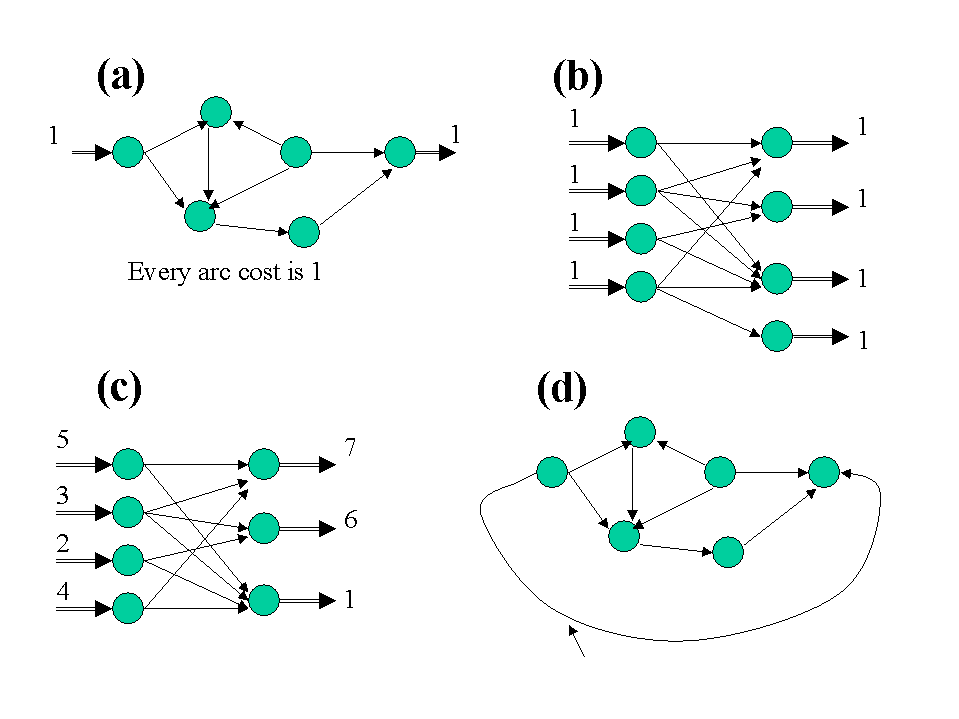This arc has cost –1;

all other arcs have cost 0

1. Assignment Problem

2. Maximum Flow Problem

3. Shortest Path Problem

4. Transportation Problem

5. None of the above (General Network Flow Problem)

(a)_____(iii)___ (b)______(i)_______

(c)_____(iv)______ (d)______(ii)______

1. (25 points) A new football stadium is being built. Each seat brings a profit of \$5000 for the team. However, to avoid traffic after the games, the state will only allow the team to build as many seats as the roads leading from the stadium to the highway can handle in one hour. For example, if the roads can handle a maximum of 75,000 people per hour, then the stadium can have up to 75,000 seats.

A network of 5 access roads exists. Currently, each road has a certain traffic capacity in each direction. No new roads can be built; however, the state will allow the football team to widen at most 3 of the access roads. Each road i has a current capacity ci, costs wi dollars to widen, and would have a capacity ki if widened.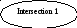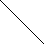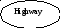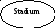Road 3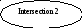1. (25 points) Formulate an integer program that the team can use to decide how many seats to put in the stadium, and which access roads (if any) it will widen in order to accommodate all of the traffic, in order to maximize its profit.

## Variables

yi = 1 if road i is widened, and 0 if not

xij = amount of traffic flow on road (i,j)

s = number of seats in stadium

## Subject to x1s + x2s – xs1 – xs2 = – s (balance at stadium)

xs1 + x21 + xh1 – x1s – x12 – x1h = 0 (balance at intersection 1)

xs2 + x12 + xh2 – x2s – x21 – x2h = 0 (balance at intersection 2)

x1h + x2h – xh1 – xh2 = s (balance at highway)
x1s c1 + (k1 – c1) y1 (capacity of each road)

xs1 c1 + (k1 – c1) y1

x2s c2 + (k2 – c2) y2

x1s c2 + (k2 – c2) y2

x12 c3 + (k3 – c3) y3

x12 c3 + (k3 – c3) y3

x1h c4 + (k4 – c4) y4

xh1 c4 + (k4 – c4) y4

x2h c5 + (k5 – c5) y5

xh2 c5 + (k5 – c5) y5
y1 + y2 + y3 + y4 + y5 3 (at most 3 roads widened)
all xij 0

all yi binary (0 or 1)

1. (Extra Credit: 5 points) Suppose the decision of which roads will be widened has already been made and cannot be changed. What special type of network problem are we left with? Why?

Maximum flow – once all the y’s are defined, this is just a max flow problem.
3. (10 points) Model each of the following using integer programming constraints. Make your constraints as tight as possible.

1. (5 points) Each week, a production manager can choose whether or not to schedule an inspector to work at his plant. Let x be the number of days the inspector works, and let y be a binary variable that is 1 if the inspector works that week, and 0 if not. Write a constraint or constraints to ensure that if the inspector is not scheduled to work, then he works zero days that week. (Note: The number of days worked can be fractional – the inspector might work 1½ days, for example.)

x  5 y (x  7 y is also okay – you could assume a 7-day work week instead of a 5-day work week)

1. (Extra Credit: 5 points) A group of friends are browsing through the local Blockbuster Video store, trying to decide which movies to rent. The friends, all ISyE professors, would like to plan their movie-watching schedule using integer programming. Let xi = 1 if movie i is rented, and 0 if not. Write a constraint or constraints to say “If we rent both The Truman Show and Pleasantville, then we can only rent one of The Matrix, Jurassic Park, and Sixty One.”

2 (xT + xP) + xM + xJ + xS 5
As you might have noticed, this one was hard. (That’s why it was extra credit!)

1. (5 points) A hardware manufacturer is going to open a new plant. Let xi = 1 if tool i will be produced there, and 0 if not. Let yj = 1 if they will buy machine j for the plant, and 0 if not. As part of the integer program the plant manager is formulating to help decide which pieces of machinery to buy, the following restriction must be converted to mathematical form: “No tools of type i can be produced unless machines A, B, and D are all bought.”

xi yA

xi yB

xi yD
This is the disaggregated form. I gave partial credit for the weaker aggregated form, 3 xi yA + yB + yD.
4. (15 points) In the following branch-and-bound tree, answer the following questions:

1. (8 points) Which nodes do you still need to branch from? Why?

Fractional node 1700 is the only one. Of all the other unbranched nodes, two are integer, one is infeasible, and fractional 2000 has a worse objective than an integer node so we know the optimal solution could never be found by branching on it.

1. (7 points) What is the gap between the best solution and the best bound found so far? Show your calculations.

Absolute gap = best integer – best bound = 1825 – 1700 = 125.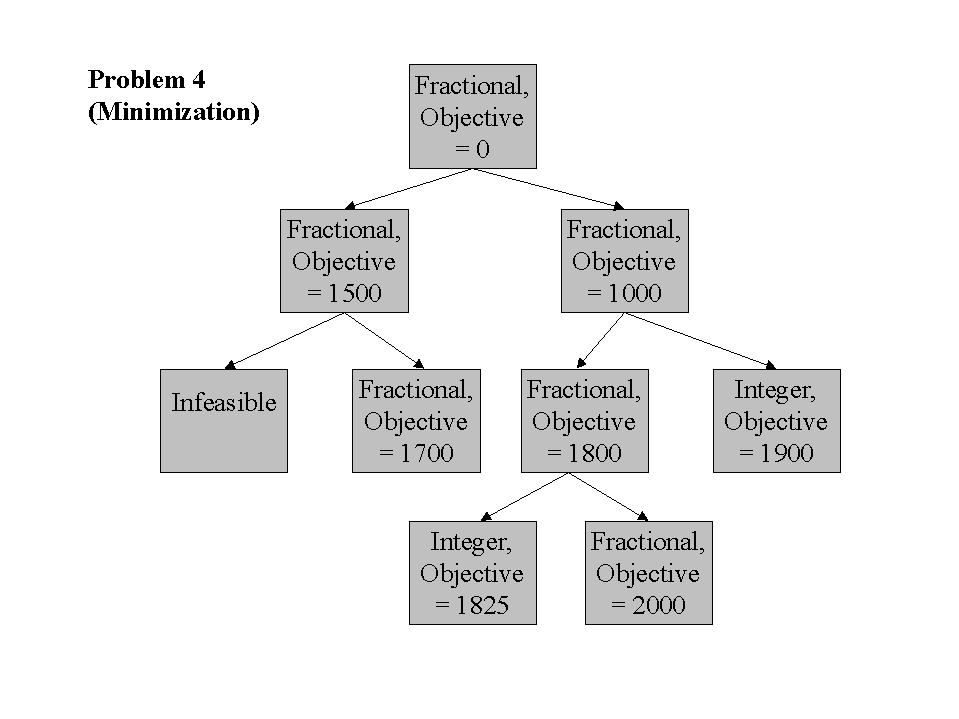5. (8 points) For the question below, select all of the correct answers from the choices given. You may need to select more than one answer per question.

Given that problem Y is NP-hard, to show that problem X is NP-hard you need to:

1. Transform problem Y into problem X in polynomial time.

2. Transform problem X into problem Y in polynomial time.

3. Show how to solve problem Y in polynomial time.

4. Show how to solve problem X in polynomial time.

(i) is the only correct answer.

1. (10 points) Answer EITHER question (a) OR question (b).

1. (10 points) Write the constraint corresponding to the LINGO statement

@FOR( origins( I):@SUM( sinks( J): x( I, J)) <= cap( I) * y ( I));

1. (10 points) Write the constraint corresponding to the AMPL statement

subject to Supply {i in origins}: sum {j in sinks} x[i,j] <= cap[i] * y[i];

j xij capi yi for all origins i

1. (16 points) Consider the following network flow problem in which the dashed arcs are the basic arcs. (The numbers shown in the figure are the costs on each arc and the supplies/demands at each node.)

7 3

(5)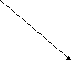(1) (3)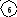(2) (8) 4

2
(7)

(4) (2)

(6)

2

1. (8 points) What are the flows on each arc at this basic solution?

(1,2)___2___ (1,3)___0___ (2,4)____3____ (2,5)___6____ (3,2)__0___

(3,5)___0___ (4,5)___0___ (4,6)____0____ (5,6)___4____
All nonbasic arcs ((1,3), (3,5), (4,5), and (4,6)) have zero flow by definition. The other flows can easily be calculated using the node balance constraints like we did in class.
(b) (8 points) What are the reduced costs of these two arcs? Show your work.

(1,2)____0_____ (3,5)____– 3 ____

Basic variables have reduced costs of zero, so (1,2) has zero reduced cost. For (3,5), find the cycle it creates among basic arcs when it is added: (3,5), (5,2), (2,3). Add costs of arcs pointing in the cycle direction (counterclockwise in this case) and subtract those in the opposite direction: 6 – 2 – 7 = – 3.